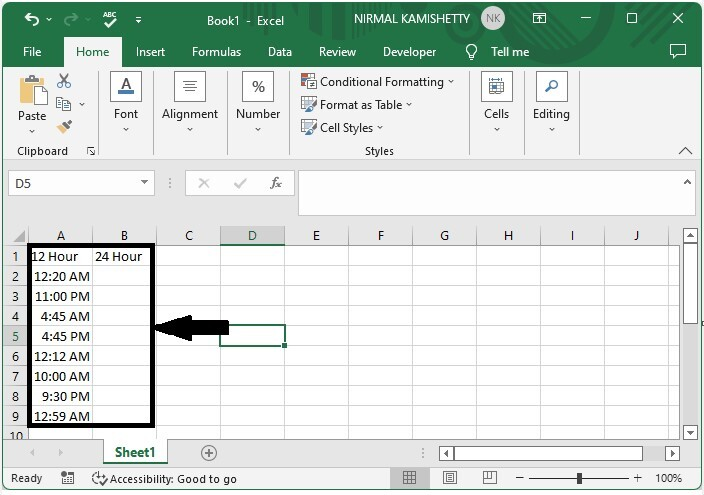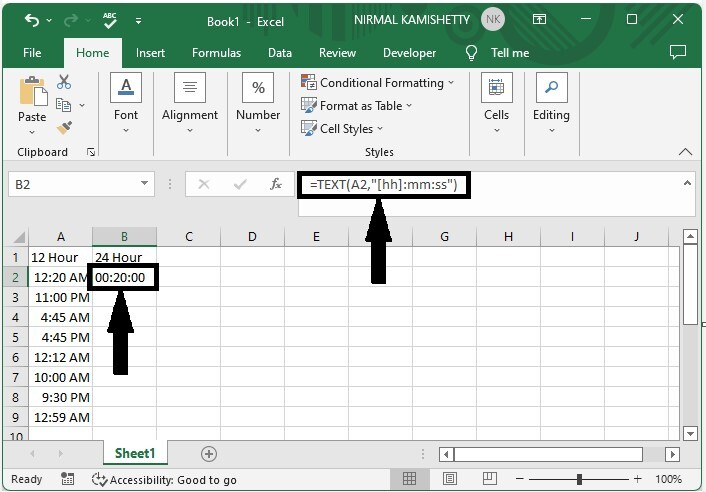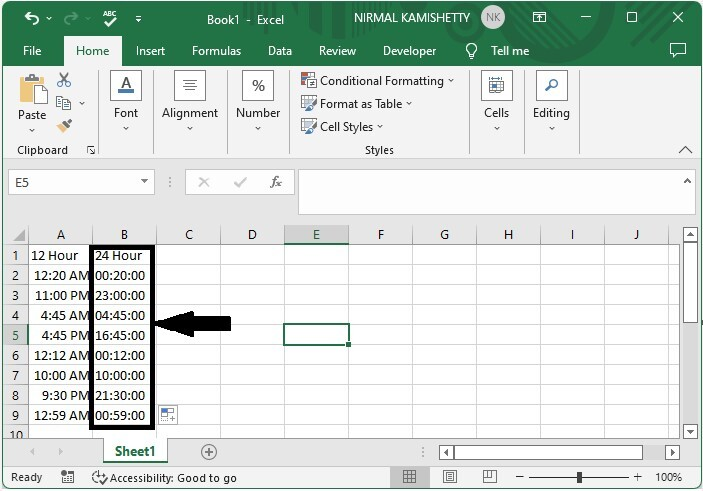# How to Convert Time Format from 12 Hour to 24 Hour and Vice Versa in Excel?

Generally, in Excel, converting time can be one of the most common tasks. If we try to do it manually, then it can be a very time-consuming and complex process. A 12-hour clock has two sets, namely AM and PM, whereas the 24-hour clock has only one set. As almost all the clocks show us the time in 12-hour format, it can be an important process to convert the time into 24-hour time because 24-hour time can be used as a standard method to use the time without mentioning am or pm. Read this tutorial to learn how you can convert time formats from 12 hour to 24 hour and vice versa in Excel.

## Converting Time Format from 12 Hour to 24 Hour in Excel

Here we will use the TEXT formula to get any one of the results, then use the autofill handle to get all the results. Let us look at a simple procedure for converting time formats from 12 hours to 24 hours in Excel. We can complete this process simply by using the formulas supported by Excel.

### Step 1

Let us consider any Excel sheet that contains a list of times in the 12-hour format as similar to the below image.Now click on the empty cell in our case, cell B2, and enter the formula as =TEXT(A2,"[hh]:mm:ss") and click Enter to get the first result as shown in the below image.### Step 2

To get all the results, drag down from the first result using the auto-fill handle, and our final result will be similar to the below image.If we need to convert 24-hour format to 12-hour format, we can use the process just by changing the formula to =TEXT(A2,"hh:mm:ss AM/Pm").

## Conclusion

In this tutorial, we used a simple example to demonstrate how you can convert time formats from 12 hour to 24 hour and vice versa in Excel.